# Rotational relaxation

(diff) ← Older revision | Latest revision (diff) | Newer revision → (diff)

Rotational relaxation refers to the decay of certain autocorrelation magnitudes related to the orientation of molecules.

If a molecule has an orientation along a unit vector n, its autocorrelation will be given by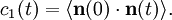$c_1(t)=\langle \mathbf{n}(0)\cdot\mathbf{n}(t) \rangle.$

From the time decay, or relaxation, of this function, one may extract a characteristic relaxation time (either from the long-time exponential decay, or from its total integral, see autocorrelation). This magnitude, which is readily computed in a simulation is not directly accessible experimentally, however. Rather, relaxation times of the second spherical harmonic are obtained: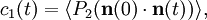$c_1(t)=\langle P_2( \mathbf{n}(0)\cdot\mathbf{n}(t) ) \rangle,$

where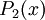$P_2(x)$ is the second Legendre polynomial.

According to simple rotational diffusion theory, the relaxation time for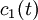$c_1(t)$ would be given by$\tau_1 = 1/2D_\mathrm{rot}$, and the relaxation time for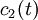$c_2(t)$ would be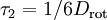$\tau_2 = 1/6D_\mathrm{rot}$. Therefore,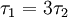$\tau_1= 3 \tau_2$. This ratio is actually lower in simulations, and closer to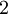$2$; the departure from a value of 3 signals rotation processes "rougher" than what is assumed in simple rotational diffusion (Ref 1).

## Water

Often, molecules are more complex geometrically and can not be described by a single orientation. In this case, several vectors should be considered, each with its own autocorrelation. E.g., typical choices for water molecules would be:

 symbol explanation experimental value, and method HH H-H axis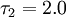$\tau_2=2.0$ps (H-H dipolar relaxation NMR) OH O-H axis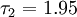$\tau_2=1.95$ps (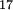$^{17}$O-H dipolar relaxation NMR)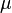$\mu$ dipolar axis not measurable, but related to bulk dielectric relaxation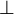$\perp$ normal to the molecule plane not measurable